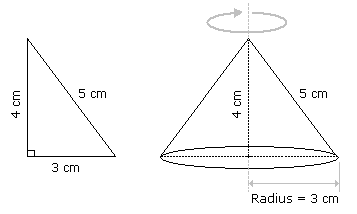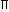7780831891 [email protected]

Instructions: This Monk test contains JK Bank Volume and Surface Problems with answer. This will help you in JK bank exam preparation.

This is a FREE online JK Bank Monk Test on topic JK Bank Volume and Surface Problems with answer. You DO NOT pay money to anyone to attend this test.
Each question carries 1 mark, no negative marks.
DO NOT refresh the page.
All the best :-).

### #1. A right triangle with sides 3 cm, 4 cm and 5 cm is rotated the side of 3 cm to form a cone. The volume of the cone so formed is:

Answer: Option A

Explanation:Clearly, we have r = 3 cm and h = 4 cm.Volume = 1r2h =1 xx 32 x 4cm3 = 12cm3. 3 3

### #2. In a shower, 5 cm of rain falls. The volume of water that falls on 1.5 hectares of ground is:

Answer: Option B

Explanation:

1 hectare = 10,000 m2

So, Area = (1.5 x 10000) m2 = 15000 m2.

 Depth = 5 m = 1 m. 100 20Volume = (Area x Depth) =15000 x 1m3 = 750 m3. 20

### #3. A hall is 15 m long and 12 m broad. If the sum of the areas of the floor and the ceiling is equal to the sum of the areas of four walls, the volume of the hall is:

Answer: Option C

Explanation:

2(15 12) x h = 2(15 x 12)h = 180 m = 20 m. 27 3Volume =15 x 12 x 20m3 = 1200 m3. 3

### #4. 66 cubic centimetres of silver is drawn into a wire 1 mm in diameter. The length of the wire in metres will be:

Answer: Option A

Explanation:

Let the length of the wire be h.

 Radius = 1 mm = 1 cm. Then, 2 2022 x 1 x 1 x h = 66. 7 20 20h =66 x 20 x 20 x 7= 8400 cm = 84 m. 22

### #5. A hollow iron pipe is 21 cm long and its external diameter is 8 cm. If the thickness of the pipe is 1 cm and iron weighs 8 g/cm3, then the weight of the pipe is:

Answer: Option B

Explanation:

External radius = 4 cm,

Internal radius = 3 cm.

Volume of iron
 =22 x [(4)2 – (3)2] x 21cm3 7
 =22 x 7 x 1 x 21cm3 7
= 462 cm3.Weight of iron = (462 x 8) gm = 3696 gm = 3.696 kg.

### #6. A boat having a length 3 m and breadth 2 m is floating on a lake. The boat sinks by 1 cm when a man gets on it. The mass of the man is:

Answer: Option B

Explanation:

 Volume of water displaced = (3 x 2 x 0.01) m3 = 0.06 m3.Mass of man = Volume of water displaced x Density of water = (0.06 x 1000) kg = 60 kg.

### #7. 50 men took a dip in a water tank 40 m long and 20 m broad on a religious day. If the average displacement of water by a man is 4 m3, then the rise in the water level in the tank will be:

Answer: Option B

Explanation:

Total volume of water displaced = (4 x 50) m3 = 200 m3.Rise in water level =200m 0.25 m = 25 cm. 40 x 20

### #8. The slant height of a right circular cone is 10 m and its height is 8 m. Find the area of its curved surface.

Answer: Option C

Explanation:

l = 10 m,

h = 8 m.

So, r = l2 – h2 = (10)2 – 82 = 6 m.Curved surface area =rl = (x 6 x 10) m2 = 60m2.

### #9. A cistern 6m long and 4 m wide contains water up to a depth of 1 m 25 cm. The total area of the wet surface is:

Answer: Option A

Explanation:

 Area of the wet surface = [2(lb   bh   lh) – lb] = 2(bh   lh)  lb = [2 (4 x 1.25 6 x 1.25) 6 x 4] m2 = 49 m2.

### #10. A metallic sheet is of rectangular shape with dimensions 48 m x 36 m. From each of its corners, a square is cut off so as to make an open box. If the length of the square is 8 m, the volume of the box (in m^3) is:

Answer: Option B

Explanation:

Clearly, l = (48 – 16)m = 32 m,

b = (36 -16)m = 20 m,

h = 8 m.Volume of the box = (32 x 20 x 8) m3 = 5120 m3.

### #11. The curved surface area of a cylindrical pillar is 264 m^2 and its volume is 924 m^3. Find the ratio of its diameter to its height.

Answer: Option B

Explanation:r2h = 924r =924 x 2= 7 m. 2rh 264 264
 And, 2rh = 264h =264 x 7 x 1 x 1= 6m. 22 2 7Required ratio = 2r = 14 = 7 : 3. h 6

### #12. A cistern of capacity 8000 litres measures externally 3.3 m by 2.6 m by 1.1 m and its walls are 5 cm thick. The thickness of the bottom is:

Answer: Option B

Explanation:

Let the thickness of the bottom be x cm.

Then, [(330 – 10) x (260 – 10) x (110 – x)] = 8000 x 1000320 x 250 x (110 – x) = 8000 x 1000(110 – x) = 8000 x 1000 = 100 320 x 250x = 10 cm = 1 dm.

### #13. What is the total surface area of a right circular cone of height 14 cm and base radius 7 cm?

Answer: Option C

Explanation:

h = 14 cm, r = 7 cm.

So, l = (7)2  (14)2 = 245 = 75 cm.Total surface arearlr2
 =22 x 7 x 75 22 x 7 x 7cm2 7 7
= [154(5  1)] cm2
= (154 x 3.236) cm2
= 498.35 cm2.

### #14. A large cube is formed from the material obtained by melting three smaller cubes of 3, 4 and 5 cm side. What is the ratio of the total surface areas of the smaller cubes and the large cube?

Answer: Option C

Explanation:

Volume of the large cube = (33  43  53) = 216 cm3.

Let the edge of the large cube be a.

So, a3 = 216a = 6 cm.Required ratio =6 x (32  42  52)= 50 = 25 : 18. 6 x 62 36

### #15. How many bricks, each measuring 25 cm x 11.25 cm x 6 cm, will be needed to build a wall of 8 m x 6 m x 22.5 cm?

Answer: Option C

Explanation:

 Number of bricks = Volume of the wall =800 x 600 x 22.5= 6400. Volume of 1 brick 25 x 11.25 x 6